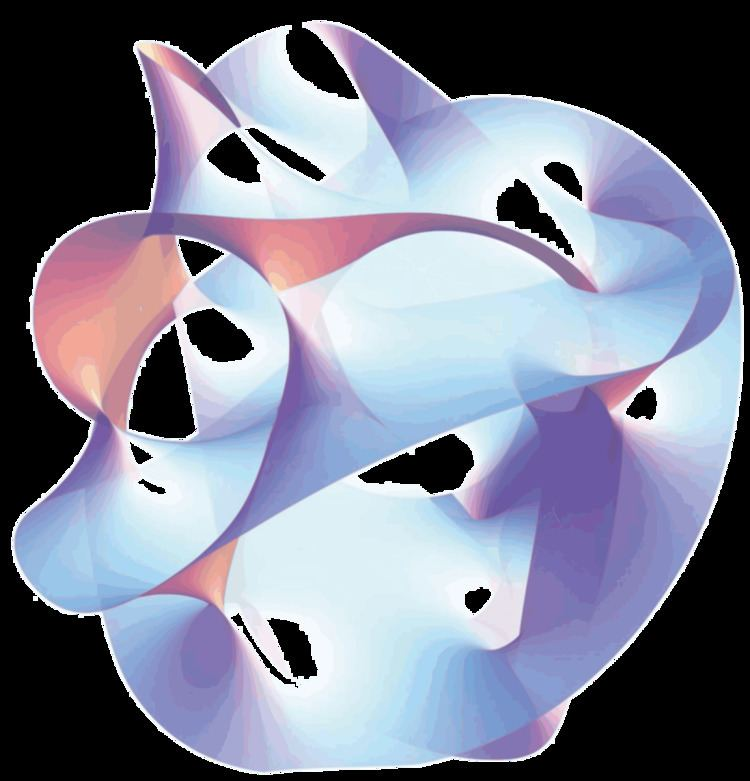# 6D (2,0) superconformal field theory

Updated on
Covid-19In theoretical physics, the six-dimensional (2,0)-superconformal field theory is a quantum field theory whose existence is predicted by arguments in string theory. It is still poorly understood because there is no known description of the theory in terms of an action functional. Despite the inherent difficulty in studying this theory, it is considered to be an interesting object for a variety of reasons, both physical and mathematical.

## Applications

The (2,0)-theory has proven to be important for studying the general properties of quantum field theories. Indeed, this theory subsumes a large number of mathematically interesting effective quantum field theories and points to new dualities relating these theories. For example, Luis Alday, Davide Gaiotto, and Yuji Tachikawa showed that by compactifying this theory on a surface, one obtains a four-dimensional quantum field theory, and there is a duality known as the AGT correspondence which relates the physics of this theory to certain physical concepts associated with the surface itself. More recently, theorists have extended these ideas to study the theories obtained by compactifying down to three dimensions.

In addition to its applications in quantum field theory, the (2,0)-theory has spawned a number of important results in pure mathematics. For example, the existence of the (2,0)-theory was used by Witten to give a "physical" explanation for a conjectural relationship in mathematics called the geometric Langlands correspondence. In subsequent work, Witten showed that the (2,0)-theory could be used to understand a concept in mathematics called Khovanov homology. Developed by Mikhail Khovanov around 2000, Khovanov homology provides a tool in knot theory, the branch of mathematics that studies and classifies the different shapes of knots. Another application of the (2,0)-theory in mathematics is the work of Davide Gaiotto, Greg Moore, and Andrew Neitzke, which used physical ideas to derive new results in hyperkähler geometry.

## References

Similar Topics
Charles Beeson (director)
Choo Hoey
Peter Gregson
Topics

 B i Link H2
 L